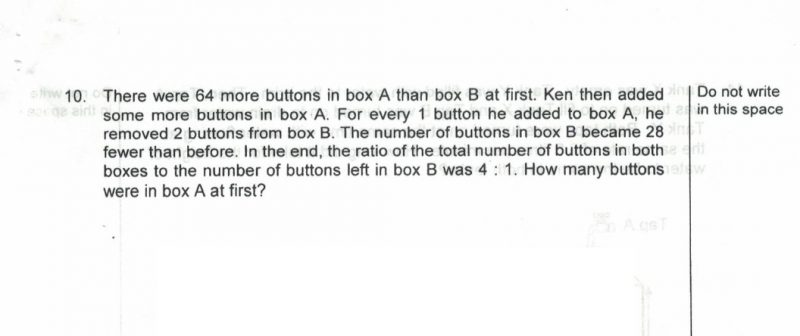# QuestionAppreciate any help provided.

thanks

1 Answer

# Answer

A= 64 more than B

For every 1 added to A , 2 removed from B

Removed from B = 28

So added to A =28/2 = 14

Now A has 106 buttons more than B (64+14+28 = 106)

Ration to total to B = 4:1

Total units 4u

B=1U           A=3u

Difference = 3-1 = 2u =106                     1 u= 106/2 =53

Number of buttons in A at first = 53×3-14 = 145

0 Replies 0 Likes